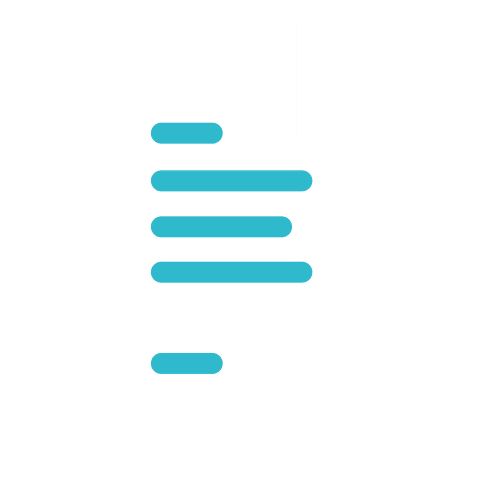# USEFUL FORMULAS & FUNCTIONS

## USEFUL FORMULAS & FUNCTIONS

### SummaryLocation

### MalaysiaDuration

2 DaysFormat

Public Class

Public Class

### Course Details

Course Code: 50449; Instructor-led

#### Audience

This course is intended for users of Microsoft Office Excel who want to learn about useful formulas and functions.

#### Prerequisites

• Basic working knowledge of Microsoft Office Excel

#### Methodology

This program will be conducted with interactive lectures, PowerPoint presentations, discussions and practical exercises

#### Course Objectives

After completing this course, students will be able to:

• Apply Formula and Functions Basic
• Statistical and Logical Functions
• Lookup and Reference Formulas
• Text Formulas
• Date and Time Formulas
• Array and Database Functions
• Efficiency Tips

### Outlines

This module explains how to understand and apply Excel basic formulas and functions.

Lessons

• Formula basics
• Using cell references
• Copy formula without changing cell reference
• Transpose formula
• Using nested functions

After completing this module, students will be able to:

• Understand and apply formula basics
• Using cell references
• Copying formula without changing cell reference
• Transpose formula using paste special
• Using nested functions

This module explains how to use logical functions including CountIf, Sumif, If, IsError.

Lessons

• Perform calculation using CountIF
• Perform calculation using SumIF
• Perform calculation using AverageA
• Using IF function to prevent division by zero
• Using IsError function to avoid error display
• Creating multiple conditions using nested IF
• Using logical function OR, And

After completing this module, students will be able to:

• Perform calculation using CountIf, SumIf, AverageA
• Using If function to prevent division by zero
• Using IsError function to avoid error display
• Create multiple conditions using nested IF
• Using logical function OR, AND

This module explains how to apply and use lookup formulas including vlookup, hlookup, match and index.

Lessons

• Using Vlookup to find specific data
• Using Hlookup to find values in rows
• Using Match and Index to retrieve data
• After completing this module, students will be able to:
• Using Vlookup to find specific data
• Using Hlookup to find values in rows
• Using Match and Index to retrieve data

This module explains how to apply Text formula to help change casing of text, append text and numerical value in excel spreadsheet.

Lessons

• Changing case of text
• Append text and numerical value
• Convert imported text format into numbers
• Break imported date field into individual columns

After completing this module, students will be able to:

• Changing case of text using Upper, Lower or Proper formula
• Append text and numerical value
• Convert imported text format into numbers
• Break imported date field into individual columns

This module explains how to make use of calculate the difference of two given Date fields and to perform calculation with Time fields.

Lessons

• Perform addition to Date fields
• Calculate difference between two Dates
• Perform calculations with Time fields

After completing this module, students will be able to:

• Perform addition and calculate difference between two dates
• Perform calculations with Time fields

This module explains how to apply and use advance formula including Array, Frequency and Database functions.

Lessons

• Using Array Formulas
• Calculate the difference between Maximum and Minimum values
• Using Frequency function to Count responses
• Using Database functions DSum and DCount

After completing this module, students will be able to:

• Using Array Formulas
• Calculating the difference between Maximum and Minimum values in an Array
• Using Frequency function to Count responses in    tabulated data
• Using Database functions DSum and DCount

This module discusses some useful Excel Tips including application of Data Validations and Auditing Tools.

Lessons

• Shortening worksheets names
• Protecting cells containing formulas
• Using Data Validation
• Displaying Formula syntax
• Using Auditing Tools for errors checking
• Tracing precedent and dependent

After completing this module, students will be able to:

• Understand the advantages of shortening worksheet names
• Protecting cells from amendments by others
• Using Data validation to improve data entries
• Using Auditing Tools for checking errors
• Adding useful notes by commenting worksheet

Interested In

Starting From
RM1000
Class Type
Private, Public

## Book Now

Course Name: USEFUL FORMULAS & FUNCTIONS
Duration: 2 Days
Class Type *
Intake Date *
First Name *
Last Name *
Email *
Contact No. *
Pax *
Total Price: RM
0.00

Course Name *
Name *
Email *
Contact No. *
Enquiry For
Company Name *
Job Position *
Message *